# ATNr

The package ATNr defines the differential equations and parametrisation of different versions of the Allometric Trophic Network (ATN) model. It is structured around a model object that contains a function implementing the ordinary differential equations (ODEs) of the model and various attributes defining the different parameters to run the ODEs.

Two different versions of the model are implemented:

• Scaled version: Delmas et al. (2017)

• Unscaled version incorporating nutrient dynamics: Schneider et al. (2016)

• Unscaled version without nutrients: Binzer et al. (2016)

The version without nutrients from Delmas et al. (2017) is scaled, meaning that the biological rates controlling the growth rate of the species are normalised by the growth rate of the smallest basal species. For more details on the three models, see the specific vignette: vignette(model_descriptions, package = "ATNr").

# A quick go through

## Creating a model

The definition of ATN is based on a model object (formally a S4 class in R). The model object is initialised specifying a fixed set of parameters: the number of species, the number of basal species, species body masses, a matrix defining the trophic interactions and, for the version including the nutrient dynamics, the number of nutrients. The first thing to do is therefore to create the corresponding R variables. While one can use an empirical food web for its analysis, it is also possible to generate synthetic food webs using the niche model from Williams and Martinez (2000) or using allometric scaling as defined in Schneider et al. (2016).

### Generating synthetic food webs (if needed)

ATNr has two functions to generate synthetic food webs, create_niche_model() for the niche model (Williams and Martinez (2000)) and create_Lmatrix() for the allometric scaling model (Schneider et al. (2016)). The niche model requires information on the number of species and connectance of the desired food web:

library(ATNr)
set.seed(123)
n_species <- 20 # number of species
conn <- 0.3 # connectance
fw <- create_niche_model(n_species, conn)

The allometric scaling model generate links based on species body masses. Therefore, it requires as an input a vector containing the body mass of species, as well as a parameter informing on the number of basal species desired. It produces a so-called L matrix which formally quantifies the probability for a consumer to successfully attack and consumer an encountered resource:

n_species <- 20
n_basal <- 5
masses <- sort(10^runif(n_species, 2, 6)) #body mass of species
L <- create_Lmatrix(masses, n_basal)

This L matrix can then be transformed into a binary food web:

fw <- L
fw[fw > 0] <- 1

More details about the generative models and the the usage precaution around them can be found in the section “The food web generative functions

### Creating a specific ATN model

As soon as a food web is stored in a matrix, it is possible to create a model object that refers to the desired specific model

# initialisation of the model object. It is possible to create a ode corresponding to
# Schneider et al. 2016, Delmas et al. 2016 or Binzer et al. 2016:
# 1) Schneider et al. 2016
n_nutrients <- 3
model_unscaled_nuts <- create_model_Unscaled_nuts(n_species, n_basal, n_nutrients, masses, fw)
# 2) Delmas et al. 2016:
model_scaled <- create_model_Scaled(n_species, n_basal, masses, fw)
# 3) Binzer et al. 2016
model_unscaled <- create_model_Unscaled(n_species, n_basal, masses, fw)

Once created, it is possible to access to the methods and attributes of the object to initialise or update them:

# updating the hill coefficient in the Unscaled_nuts model:
model_unscaled_nuts$q <- 1.4 # Changing the assimilation efficiencies of all species to 0.5 in the Scaled model: model_scaled$e = rep(0.5, model_scaled$nb_s) # print the different fields that can be updated and their values: # str(model_unscaled_nuts) It is important to keep in mind that some rules apply here: • The order of the species in the different fields must be consistent: the first species in the $BM object corresponds to the first species in the $fw object and in the $e object.

• The objects that are specific to a species type (i.e. basal species or consumers) are dimensioned accordingly: the handling time ($h) sets the handling time of consumers on resources. Therefore, the h matrix has a number of rows equal to the number of species and a number of columns equal to the number of consumers (as non consumer species do not have a handling time by definition). In that case, the first row correspond to the first species and the first column to the first consumer. • The object describing the interactions between plants and nutrients ($K or $V) are matrices for which the number of rows equals to the number of nutrients and a number of columns matches the number of basal species (this point is specific to the Schneider model which is the only one including an explicit dynamics of the nutrient pool). To run the population dynamics, all the parameters must be defined. It is possible to automatically load a by default parametrisation using the dedicated functions: # for a model created by create_model_Unscaled_nuts(): model_unscaled_nuts <- initialise_default_Unscaled_nuts(model_unscaled_nuts, L) # for a model created by create_model_Scaled(): model_scaled <- initialise_default_Scaled(model_scaled) # for a model created by create_model_Unscaled(): model_unscaled <- initialise_default_Unscaled(model_unscaled) Importantly, for the unscaled with nutrients model of Schneider et al. (2016), the calculation of consumption rates rely on the L matrix created above or, in case of empirical networks, by a matrix that defines the probability of a consumer to successfully attack and consume an encountered prey. The default initialisation of the Unsacled_nuts and Unscaled models can also include temperature effects (20° C by default). ## Running the population dynamics Once all the parameters are properly defined, the ODEs can be integrated by any solver. We present here a solution based on lsoda from library deSolve (Soetaert, Petzoldt, and Setzer (2010)), but other solutions exist (sundialr is also a possibility). The package propose a direct wrapper to lsoda with the function lsoda_wrapper: biomasses <- masses ^ -0.75 * 1e1 # starting biomasses biomasses <- append(runif(3, 20, 30), biomasses) # nutrient concentration # defining the desired integration time times <- seq(0, 1500, 5) sol <- lsoda_wrapper(times, biomasses, model_unscaled_nuts) To have more control of the integration, it is however possible to not use the wrapper proposed in the package and directly work with the lsoda function. Here is an example: # running simulations for the Schneider model model_unscaled_nuts$initialisations()
sol <- deSolve::lsoda(
biomasses,
times,
function(t, y, params) {
return(list(params$ODE(y, t))) }, model_unscaled_nuts ) Not that the call of model_unscaled_nuts$initialisation() is important here as it pre-computes some variables to optimise code execution. This function is normally internally called by lsoda_wrapper. In case of an integration that does not rely on this wrapper function, the call to $initialisation() is needed for ALL the model types. The package also contains a simple function to plot the time series obtained: plot_odeweb. par(mar = c(4, 4, 1, 1)) plot_odeweb(sol, model_unscaled_nuts$nb_s)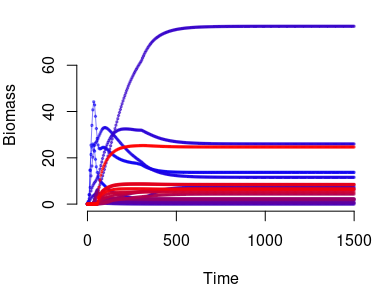# The food web generative functions

It is possible to create a model object using empirical food webs, however synthetic ones can be valuable tools to explore different theoretical questions. To allow this possibility, two different models are available in the package: the niche model (Williams and Martinez (2000)) or the allometric scaling model (Schneider et al. (2016)). Thereafter, we use the following function to visualise the adjacency matrices (where rows correspond to resources and columns to consumers) of the food webs:

# function to plot the fw
show_fw <- function(mat, title = NULL) {
par(mar = c(.5, .5, 2, .5))
S <- nrow(mat)
mat <- mat[nrow(mat):1, ]
mat <- t(mat)
image(mat, col = c("goldenrod", "steelblue"),
frame = FALSE, axes = FALSE)
title(title)
grid(nx = S, ny = S, lty = 1, col = adjustcolor("grey20", alpha.f = .1))
}

The niche model orders species based on their trophic niche, randomly sampled from a uniform distribution. For each species $$i$$, a diet range ($$r_i$$) is then drawn from a Beta distribution and a diet center $$c_i$$ from a uniform distribution. For each species $$i$$, all species that have trophic niche within the interval $$[c_i - r_i / 2, c_i + r_i / 2]$$ are considered to be prey of species $$i$$. In this package, we followed the modification to the niche model of Williams and Martinez (2000) as specified in Allesina, Alonso, and Pascual (2008).

Generating a food web from the niche model is made by a simple call to the corresponding functions:

S <- 50 # number of species
C <- 0.2 # connectance
fw <- create_niche_model(S, C)

The function ensure that the food web returned are not composed of disconnected sub networks (i.i several connected components).

The allometric scaling model assumes an optimal consumer/resource body mass ratio (Ropt, default = 100) for attack rates, i.e. the probability that when a consumer encounter a species it will predate on it. In particular, each attack rate is calculated using a Ricker function:

$a_{ij} = \left( \frac{m_i}{m_j \cdot Ropt} \cdot e^{(1 - \frac{m_i}{m_j \cdot Ropt})} \right) ^\gamma$

where $$m_i$$ is the body mass of species $$i$$ and $$\gamma$$ sets the width of the trophic niche.

Generating a food web with the allometric scaling model necessitate few more steps. The trophic niche of species is defined by a body mass interval and is quantified (see fig. 2 and 3 from Schneider et al., 2016). This quantified version actually return the probabilities of a successful attack event to occur when a consumer encounter a prey. These probabilities are estimated with a Ricker function of 4 parameters: the body masses of the resource and of the consumer, the optimal predator-prey body mass ratio Ropt and the width of the trophic niche gamma. A threshold (th) filters out links with very low probabilities of attack success. The probabilities are stored in a matrix obtained from:

# number of species and body masses
n_species <- 20
n_basal <- 5
# body mass of species. Here we assume two specific rules for basal and non basal species
masses <- c(sort(10^runif(n_basal, 1, 3)), sort(10^runif(n_species - n_basal, 2, 6)))
L <- create_Lmatrix(masses, n_basal, Ropt = 100, gamma = 2, th = 0.01)

Then, a food web is a binary version of the L matrix that can be stored either using booleans (FALSE/TRUE) or numeric values (0/1):

# boolean version
fw <- L > 0
# 0/1 version:
fw <- L
fw[fw > 0] <- 1
show_fw(fw, title = "L-matrix model food web")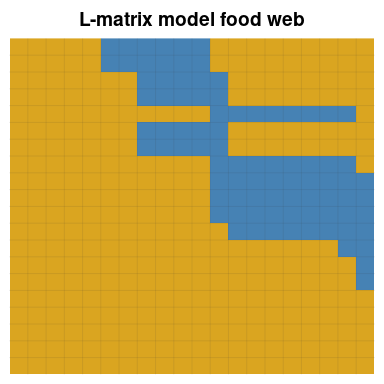# Examples

## effect of temperature on species persistence

ATNr makes it relatively easy to vary one parameter to assess its effect on the population dynamics. For example, we can study how changes in temperatures from 15 to 30 Celsius degrees affects the number species to go extinct.

set.seed(12)
# 1) define number of species, their body masses, and the structure of the
# community
n_species <- 50
n_basal <- 20
n_nut <- 2
# body mass of species
masses <- 10 ^ c(sort(runif(n_basal, 1, 3)),
sort(runif(n_species - n_basal, 2, 9)))
# 2) create the food web
# create the L matrix
L <- create_Lmatrix(masses, n_basal, Ropt = 50, gamma = 2, th = 0.01)
# create the 0/1 version of the food web
fw <- L
fw[fw > 0] <- 1
# 3) create the model
model <- create_model_Unscaled_nuts(n_species, n_basal, n_nut, masses, fw)
# 4) define the temperature gradient and initial conditions
temperatures <- seq(4, 22, by = 2)
extinctions <- rep(NA, length(temperatures))
# defining biomasses
biomasses <- runif(n_species + n_nut, 2, 3)
# 5) define the desired integration time.
times <- seq(0, 100000, 100)
# 6) and loop over temperature to run the population dynamics
i <- 0
for (t in temperatures){
# initialise the model parameters for the specific temperature
# Here, no key parameters (numbers of species or species' body masses) are modified
# Therefore, it is not needed to create a new model object
# TO reinitialise the different parameters is enough
model <- initialise_default_Unscaled_nuts(model, L, temperature = t)
model$q <- 1.2 model$S <- rep(10, n_nut)
# running simulations for the Schneider model:
sol <- lsoda_wrapper(times, biomasses, model, verbose = FALSE)
# retrieve the number of species that went extinct before the end of the
# simulation excluding here the 3 first columns: first is time, 2nd and 3rd
# are nutrients
i <- i + 1
extinctions[i] <- sum(sol[nrow(sol), 4:ncol(sol)] < 1e-6)
}
plot(temperatures, extinctions,
pch = 20, cex = 0.5, ylim = c(0,50), frame = FALSE,
ylab = "Number of Extinctions", xlab = "Temperature (°C)")
lines(temperatures, extinctions, col = 'blue')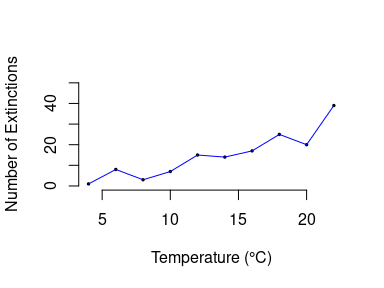## Effect of predator-prey body mass ratio and temperature on species persistence

Predator-prey body mass ratio and environment temperature have been shown to affect persistence of species in local communities, e.g. Binzer et al. (2016). Here, we use the ATNr model (name here) to replicate the results from Binzer et al. (2016). In particular, we compute the fraction of species species that persist for predator-prey body mass ratio values in $$\left[ 10^{-1}, 10^4 \right]$$ and temperature values in $$\{0, 40\}$$ °C.

First, we create a food web with 30 species and initialize within a for loop the model with a given value of body mass ratio and temperature. Species persistence is calculate as the fraction of species that are not extinct at the end of the simulations.

# set.seed(142)

# number of species
S <- 30

# vector containing the predator prey body mass ratios to test
scaling <- 10 ^ seq(-1, 4, by = .5)

# vectors to store the results
persistence0 <- c()
persistence40 <- c()

# create the studied food web
fw <- create_niche_model(S = S, C = 0.1)
# calculating trophic levels
TL = TroLev(fw)
biomasses <- runif(S, 2, 3)

# run a loop over the different pred-prey body mass ratios
for (scal in scaling) {
# update species body masses following the specific body mass ratio
masses <- 0.01 * scal ^ (TL - 1)

# create the models with parameters corresponding to 0 and 40 degrees Celcius
mod0 <- create_model_Unscaled(nb_s = S,
nb_b = sum(colSums(fw) == 0),
BM = masses,
fw = fw)
mod0 <- initialise_default_Unscaled(mod0, temperature = 0)
mod0$c <- rep(0, mod0$nb_s - mod0$nb_b) mod0$alpha <- diag(mod0$nb_b) mod40 <- create_model_Unscaled(nb_s = S, nb_b = sum(colSums(fw) == 0), BM = masses, fw = fw) mod40 <- initialise_default_Unscaled(mod40, temperature = 40) mod40$c <- rep(0, mod40$nb_s - mod40$nb_b)
mod40$alpha <- diag(mod40$nb_b)

times <- seq(1, 1e9, by = 1e7)

# run the model corresponding to the 0 degree conditions
sol <- lsoda_wrapper(times, biomasses, mod0, verbose = FALSE)
persistence0 <- append(persistence0, sum(sol[nrow(sol), -1] > mod0$ext) / S) # run the model corresponding to the 40 degrees conditions sol <- lsoda_wrapper(times, biomasses, mod40, verbose = FALSE) persistence40 <- append(persistence40, sum(sol[nrow(sol), -1] > mod40$ext) / S)
}

Similarly to Binzer et al. (2016), species persistence increases with increasing values of predator-prey body mass ratios, but temperature effect differs depending n this ratio: when predator prey body mass ratio is low, high temperature lead to more persistence while increasing predator prey body mass ratio tend to reduce the effects of temperature.

plot(log10(scaling), persistence40,
xlab = expression("Body mass ratio between TL"[i + 1]* " and TL"[i]),
ylab = "Persistence",
ylim = c(0, 1),
frame = FALSE, axes = FALSE, type = 'l', col = "red")
lines(log10(scaling), persistence0, col = "blue")
axis(2, at = seq(0, 1, by = .1), labels = seq(0, 1, by = .1))
axis(1, at = seq(-1, 4, by = 1), labels = 10 ^ seq(-1, 4, by = 1))
legend(0.1, 0.9, legend = c("40 \u00B0C", "0 \u00B0C"), fill = c("red", "blue"))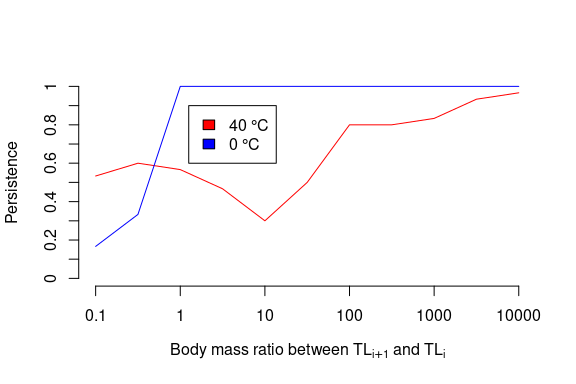The paradox of enrichment states that by increasing the carrying capacity of basal species may destabilize the population dynamics Rosenzweig (1971). Here, we show how this can be studied with the ATNr; we used the model from Delmas et al. (2017), but similar results can be obtained using the other two models in the package.

First, we create a food web with 20 species and initialize the model

set.seed(1234)
S <- 10
fw <- NULL
TL <- NULL
fw <- create_niche_model(S, C = .15)
TL <- TroLev(fw)

masses <- 0.01 * 100 ^ (TL - 1) #body mass of species

mod <- create_model_Scaled(nb_s = S,
nb_b = sum(colSums(fw) == 0),
BM = masses,
fw = fw)
mod <- initialise_default_Scaled(mod)
times <- seq(0, 500, by = 2)
biomasses <- runif(S, 2, 3) # starting biomasses

Then, we solve the system specifying the carrying capacity of basal species equal to one (mod$K <- 1) and then increased this to ten (mod$K <- 10)

mod$K <- 1 sol1 <- lsoda_wrapper(times, biomasses, mod, verbose = FALSE) mod$K <- 10
sol10 <- lsoda_wrapper(times, biomasses, mod, verbose = FALSE)

As shown in the plot below, for K = 1 the system reaches a stable equilibrium, whereas when we increase the carrying capacity (K = 10) the system departs from this stable equilibrium and periodic oscillations appear.

par(mfrow = c(2, 1))
plot_odeweb(sol1, S)
title("Carrying capacity = 1")
plot_odeweb(sol10, S)
title("Carrying capacity = 10")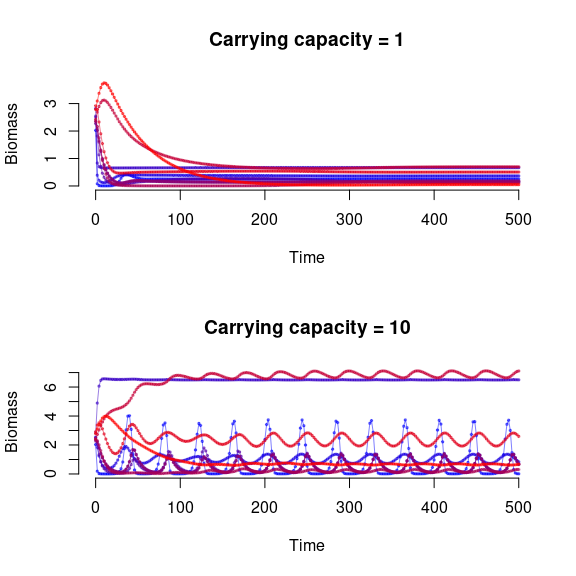# Common mistakes, things to not do

## Not updating model parameters properly in the model object

The building block of this package are the C++ classes to solve ODEs for the ATN model. Model parameters are stored in such classes and can be changed only by addressing them within the respective objects. For instance:

set.seed(1234)
nb_s <- 20
nb_b <- 5
nb_n <- 2
masses <- sort(10 ^ runif(nb_s, 2, 6)) #body mass of species
biomasses = runif(nb_s + nb_n, 2, 3)
L <- create_Lmatrix(masses, nb_b, Ropt = 50)
L[, 1:nb_b] <- 0
fw <- L
fw[fw > 0] <- 1
model_unscaled_nuts <- create_model_Unscaled_nuts(nb_s, nb_b, nb_n, masses, fw)
model_unscaled_nuts <- initialise_default_Unscaled_nuts(model_unscaled_nuts, L)
nb_s <- 30 #this does not change the model parameter
model_unscaled_nuts$nb_s #this is the model parameter #>  20 ## Updating key parameters without creating a new model object Changing parameters that are used in the create_model functions without creating a new model object is almost always a bad idea. Those parameters are important structural parameters, and changing one of them implies changes in most of the other variables contained in the model object. For instance, the example above, changing the number of species in the model object will lead to inconsistencies in the different variables: the dimensions of objects storing attack rates, body masses and so on won’t match the updated number of species. Some basic checks are made before starting the integration in the lsoda_wrapper function, based on the run_checks procedure also available in the package. times <- seq(0, 15000, 150) model_unscaled_nuts$nb_s = 40
# this will return an error :
# sol <- lsoda_wrapper(times, biomasses, model_schneider)

However, some modification can remain undetected. For instance, modifying species’ body masses only won’t raise any errors. However, a change in species body mass should be associated to a change in all the associated biological rates. The following code won’t raise any errors, but will produce results relying on a model with an incoherent set of parameters and therefore wrong result:

set.seed(1234)
nb_s <- 20
nb_b <- 5
nb_n <- 2
masses <- sort(10 ^ runif(nb_s, 2, 6)) #body mass of species
biomasses = runif(nb_s + nb_n, 2, 3)
L <- create_Lmatrix(masses, nb_b, Ropt = 50)
L[, 1:nb_b] <- 0
fw <- L
fw[fw > 0] <- 1
model_unscaled_nuts <- create_model_Unscaled_nuts(nb_s, nb_b, nb_n, masses, fw)
model_unscaled_nuts <- initialise_default_Unscaled_nuts(model_unscaled_nuts, L)
model_unscaled_nuts$BM <- sqrt(model_unscaled_nuts$BM) # we change body masses within the model
sol <- lsoda_wrapper(seq(1, 5000, 50), biomasses, model_unscaled_nuts)
par(mar = c(4, 4, 1, 1))
plot_odeweb(sol, model_unscaled_nuts$nb_s)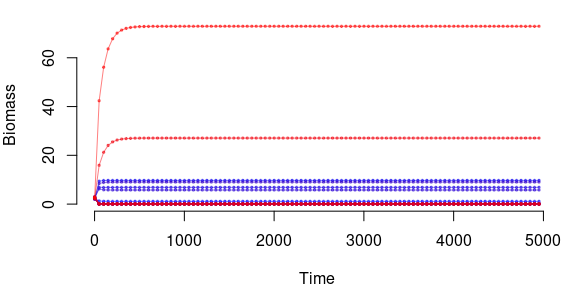In general, each time one of these key parameters is modified (nb_s, nb_b, nb_n for the Schneider model, BM, fw), it is strongly recommended to create a new model objects with the updated parameters: nb_s <- 30 nb_n <- 2 masses <- sort(10 ^ runif(nb_s, 2, 6)) #body mass of species biomasses <- runif(nb_s + nb_n, 2, 3) L <- create_Lmatrix(masses, nb_b, Ropt = 50) L[, 1:nb_b] <- 0 fw <- L fw[fw > 0] <- 1 # create a new object: model_unscaled_nuts <- create_model_Unscaled_nuts(nb_s, nb_b, nb_n, masses, fw) model_unscaled_nuts <- initialise_default_Unscaled_nuts(model_unscaled_nuts, L) # safely run the integration: sol <- lsoda_wrapper(times, biomasses, model_unscaled_nuts) Specifically to the Schneider model, changing the ‘Lmatrix’ requires to update the feeding rate ($b).

Changing the dimensions of a vector or matrix object somehow implies a change in the number of species (see section above). For instance, decreasing the length of the assimilation efficiencies vector $e should imply a consistent update of all the other parameters (handling times, attack rates, body masses, etc depending on the model). The function remove_species is made to properly remove species from model objects without having to manually regenerate all parameters. ## copying model to another model variable As built on Rcpp, the different models are only pointers to C++ objects. It means that this script: nb_s <- 30 nb_n <- 2 masses <- sort(10 ^ runif(nb_s, 2, 6)) #body mass of species biomasses <- runif(nb_s + nb_n, 2, 3) L <- create_Lmatrix(masses, nb_b, Ropt = 50) L[, 1:nb_b] <- 0 fw <- L fw[fw > 0] <- 1 # create a new object: model_1 <- create_model_Unscaled_nuts(nb_s, nb_b, nb_n, masses, fw) model_1 <- initialise_default_Unscaled_nuts(model_1, L) # trying to create a new model that is similar to model_1 model_2 = model_1 will not create a new model object. Formally, it creates a new pointer to the same address, which means that model_1 and model_2 are in reality the same variable (shallow copy). Therefore, modifying one modifies the other: model_1$q = 1.8
# this also updated the value in model_2:
model_2\$q
#>  1.8

Therefore, to create a new model object based on another one, it is important to formally create one (either with one of the create_model_ function, or using new). More information on copying variables using pointers here: https://stackoverflow.com/questions/184710/what-is-the-difference-between-a-deep-copy-and-a-shallow-copy

Note: Should we let the possibility for users to update these key parameters (i.e. the ones in the create_model() functions)? Should we restrict them that they can only be read and not modified? For instance by not exporting them and creating a get_x() method that would return the value of x? This options is much better in terms of “security” but much less straightforward to use.

Allesina, Stefano, David Alonso, and Mercedes Pascual. 2008. “A General Model for Food Web Structure.” Science 320 (5876): 658–61.
Binzer, Amrei, Christian Guill, Björn C Rall, and Ulrich Brose. 2016. “Interactive Effects of Warming, Eutrophication and Size Structure: Impacts on Biodiversity and Food-Web Structure.” Global Change Biology 22 (1): 220–27.
Delmas, Eva, Ulrich Brose, Dominique Gravel, Daniel B Stouffer, and Timothée Poisot. 2017. “Simulations of Biomass Dynamics in Community Food Webs.” Methods in Ecology and Evolution 8 (7): 881–86.
Rosenzweig, Michael L. 1971. “Paradox of Enrichment: Destabilization of Exploitation Ecosystems in Ecological Time.” Science 171 (3969): 385–87. https://doi.org/10.1126/science.171.3969.385.
Schneider, Florian D, Ulrich Brose, Björn C Rall, and Christian Guill. 2016. “Animal Diversity and Ecosystem Functioning in Dynamic Food Webs.” Nature Communications 7 (1): 1–8.
Soetaert, Karline, Thomas Petzoldt, and R. Woodrow Setzer. 2010. “Solving Differential Equations in R: Package deSolve.” Journal of Statistical Software 33 (9): 1–25. https://doi.org/10.18637/jss.v033.i09.
Williams, Richard J, and Neo D Martinez. 2000. “Simple Rules Yield Complex Food Webs.” Nature 404 (6774): 180–83.